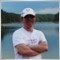# Trying to pass object to class from CArrayObj Object need help in understanding what I am doing wrong119

Hello Everyone,

I am looking for some help with the below code, I am trying to pass an object to a different class from a CArrayObj class using the At method which returns an object.

But so far everything I have tried gives a critical crash stating access to an invalid pointer. So how can I make this code work, what am I missing?

EK

```//+------------------------------------------------------------------+
//|                                                        Test2.mq5 |
//|                        Copyright 2010, MetaQuotes Software Corp. |
//|                                              http://www.mql5.com |
//+------------------------------------------------------------------+
#property version   "1.00"
#property indicator_chart_window

#include <Object.mqh>
//#include <Arrays\ArrayObj.mgh>

class A : public CObject
{
public:
string test_str;
int test_int;
float test_float;

// constructor
void A()    {test_str = "0"; test_int = 0; test_float = 0.0;}
// function
void Set_Values(A *nNew);

};

void A::Set_Values(A *nNew)
{
test_str = nNew.test_str;
}

class B
{
public:
A *MyA; // my instance of Cass A

// constructor
void B()    { MyA = new A; }

//function
void Set_MyObject(A *NewObj);

};

void B::Set_MyObject(A *NewObj)
{
MyA.test_str = NewObj.test_str;
MyA.test_int = NewObj.test_int;
MyA.test_float = NewObj.test_float;
}

CArrayObj *MyListOfObjects;
B *ThisIsMyB;

//+------------------------------------------------------------------+
//| Custom indicator initialization function                         |
//+------------------------------------------------------------------+
int OnInit()
{
int i;

// Create 10 new Objects of Class A and puts them in our ArrayObj List
for(i = 0; i<10; i++)
{
A *Temp = new A;
}

// now lets say I wanted to make  one from my list and give it to Class B
// ** but this gives invalid pointer access ***
ThisIsMyB.Set_MyObject(MyListOfObjects.At(7));

return(0);
}
//+------------------------------------------------------------------+
//| Custom indicator iteration function                              |
//+------------------------------------------------------------------+
int OnCalculate(const int rates_total,
const int prev_calculated,
const datetime& time[],
const double& open[],
const double& high[],
const double& low[],
const double& close[],
const long& tick_volume[],
const long& volume[],
{
//---
//--- return value of prev_calculated for next call
return(rates_total);
}
//+------------------------------------------------------------------+
```
Documentation on MQL5: MQL5 programs / Runtime Errors
• www.mql5.com
MQL5 programs / Runtime Errors - Documentation on MQL516974

Where is

```MyListOfObjects =new CArrayObj;
```

?119

Rosh:

Where is

?

Hello Rosh,

Thank you that helped a lot, I was able to track my problem down :)

Thanks

EK